﻿ Identifying Conics by the Discriminant

# Identifying Conics by the Discriminant

• PRACTICE (online exercises and printable worksheets)

Conic sections—circles/ellipses, parabolas, hyperbolas—were introduced in the prior section.
They arise by intersecting a plane with the surface of an infinite double cone.
In this current section, we begin the analysis of equations representing conic sections.

There are results in this section for which you might be wondering: ‘Why is this true?’
If so, then you're encouraged to study the following optional sections:

## General Conic Equation

Let $\,A\,$, $\,B\,$, $\,C\,$, $\,D\,$, $\,E\,$ and $\,F\,$ be real numbers. Consider the equation: $$Ax^2 + Bxy + Cy^2 + Dx + Ey + F = 0 \quad (\,\dagger\,)$$

The $\,x^2\,$, $\,xy\,$ and $\,y^2\,$ terms are often called the degree-two terms in this equation.
Why? Because the exponents are $\,2\,$, or they add to two: $$\,xy = x^1y^1\ \ \ \ \text{ and } \ \ \ \ 1 + 1 = 2$$

There is a beautiful relationship between equations of this type and the graphs of conic sections!
Indeed, both of the following are true:

• Given any equation of the form ($\,\dagger\,$), it graphs as a conic, a degenerate conic,
or a curve that arises from a ‘limiting case’ of an infinite double cone (discussed below).
• Given the graph of any conic section, drawn anywhere in an $\,xy\,$-plane, it can be described by an equation of the form ($\,\dagger\,$).
For this reason, ($\,\dagger\,$) is called the general conic equation.
If the (nonzero) terms are in precisely the same order as indicated in ($\,\dagger\,$),
with zero on the right-hand side, then the general conic equation is said to be in standard form.
(By the way, the symbol ‘$\,\dagger\,$’ is a ‘dagger’, and is often used to mark an important equation.)

When you're ‘on the lookout’ for conics, you certainly don't need the equation to be in standard form!
Just check that there are no term types other than $\,x^2\,$, $\,xy\,$, $\,y^2\,$, $\,x\,$, $\,y\,$ and constants.
Any of the coefficients can equal zero, so you might not have all these term types.

It's not particularly easy to go from an arbitrary equation of the form ($\,\dagger\,$) to its graph, using paper-and-pencil.
(In the next few sections, you'll learn to graph fairly simple conics.)
However, WolframAlpha is up to the task!
If you're bored, jump up there, type in a random general conic equation, and see the awesomeness of WolframAlpha!

Here are some WolframAlpha results to illustrate the variety of conic sections (including some degenerate and limiting cases).
You can cut-and-paste the equations below each graphic into WolframAlpha yourself to verify the results. Enjoy!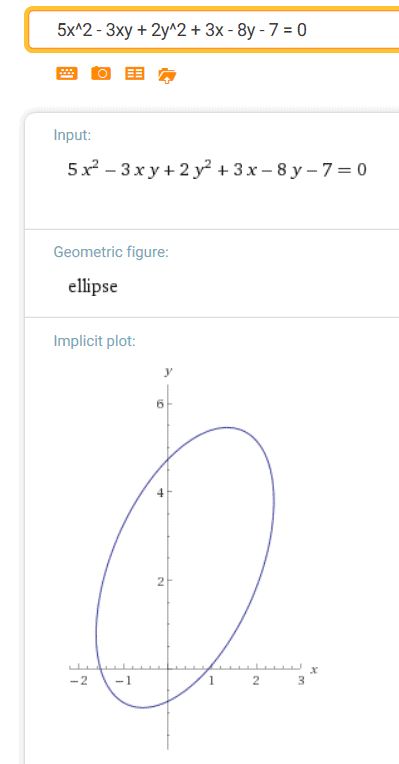5x^2-3xy+2y^2+3x-8y-7 = 0(x-1)^2 + (y+3)^2 = 16xy = 1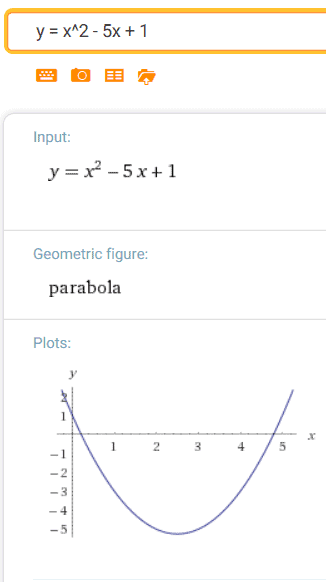y = x^2 - 5x + 1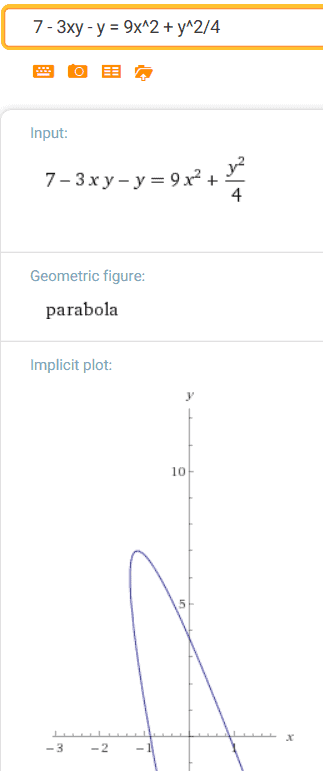7 - 3xy - y = 9x^2 + y^2/4x^2 + 2xy + y^2 = 0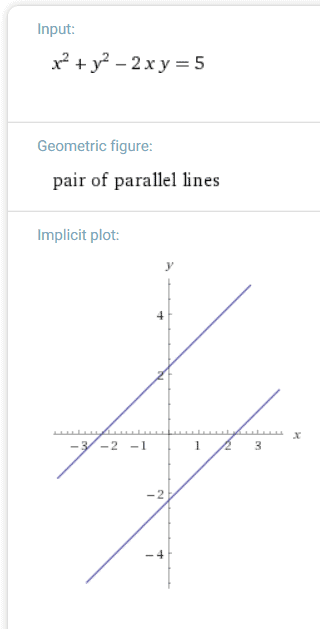x^2 + y^2 - 2xy = 5x^2 - y^2 = 0 

Or, you can play with the equation $\,Ax^2 + Bxy + Cy^2 + Dx + Ey + F = 0\,$ below.
Use the sliders to set the desired values for the parameters $\,A\,$, $\,B\,$, $\,C\,$, $\,D\,$, $\,E\,$, and $\,F\,$.
The initial configuration is $\,5x^2 - 3xy + 2y^2 + 3x - 8y - 7 = 0\,$; refresh the page to reset.
You can use the navigation arrows at the bottom right to see more of the graph.
Enjoy!

## Conic Discriminant

Even though conics can be challenging to graph, here's some really good news.
There's a simple way to decide which type of conic is represented by a given general conic equation, as follows:

• Read off the coefficients $\,A\,$, $\,B\,$ and $\,C\,$ from ($\,\dagger\,$).
Note: As long as all the degree-two terms are on the same side of the equation, then:
• $A\,$ is the coefficient of the $\,x^2\,$ term
• $B\,$ is the coefficient of the $\,xy\,$ term
• $C\,$ is the coefficient of the $\,y^2\,$ term
• Compute $\,B^2 - 4AC\,$, which we'll call the conic discriminant.
(In context, it will more simply be called the discriminant.)
• Use the table below to determine the type of conic represented by the equation:

 CONIC DISCRIMINANT TYPE OF CONIC SECTION POSSIBLE MEMORY DEVICE $B^2 - 4AC < 0$ ELLIPSE (possibly degenerate) If, in addition, $\,A = C\,$ then it's a circle. Look at the letters in the word ELLIPSE! There's an L. There's an E. There's an S. It practically spells out ‘less’! When the discriminant is less than zero, it's an ellipse. $B^2 - 4AC = 0$ PARABOLA (possibly degenerate) (no memory device) $B^2 - 4AC > 0$ HYPERBOLA (possibly degenerate) If you're HYPER, then you have a GREAT deal of energy. When the discriminant is GREATer than zero, it's a HYPERbola.
The expression $\,B^2-4AC\,$ is called the discriminant because it helps us discriminate (differentiate between) the different types of conics.

Note the similarity to the discriminant of a quadratic equation $\,ax^2 + bx + c = 0\,$.
The quadratic discriminant is $\,b^2 - 4ac\,$, and helps us decide how many real solutions the quadratic equation has.

## EXAMPLES: Using the Discriminant to Determine the Type of Conic

Example 1: general conic equation in standard form

Consider the equation $\,5x^2 - 3xy + 2y^2 + 3x - 8y - 7 = 0\,$ (from the WolframAlpha example above).
It's already in the form $\,Ax^2 + Bxy + Cy^2 + Dx + Ey + F = 0\,$, so just read off the required coefficients $$\begin{gather} A = 5\cr B = -3\cr C = 2\cr\cr \text{and compute the discriminant}\cr\cr B^2 - 4AC \ =\ (-3)^2 - 4(5)(2) \ =\ -31 \ < \ 0 \end{gather}$$ The discriminant is less than zero—it's an ellipse.

Example 2: general conic equation not in standard form

Consider the equation $\,y = x^2 - 5x + 1\,$.
It's a general conic equation, but it's not in standard form.

From prior mathematical experience, you know that quadratic functions graph as parabolas.
However, let's check that the ‘conic discriminant’ gives the same information.

You could put the equation in standard form: either $$x^2 + 0xy + 0y^2 - 5x - y + 1 = 0$$ or $$-x^2 + 0xy + 0y^2 + 5x + y - 1 = 0$$ In the first way, you'd read off $\,A = 1\,$ and $\,B = C = 0\,$.
In the second way, you'd read off $\,A = -1\,$ and $\,B = C = 0\,$.

But, there's an easier thought process.
Just check that the degree-two terms are all on the same side of the equation.
For the equation $\,y = x^2 - 5x + 1\,$, there's only one degree-two term ($\,x^2\,$), so the side it's on doesn't matter.
So, $\,A = 1\,$ and $\,B = C = 0\,$.

In all cases, $\,B^2 - 4AC = 0\,$.
The discriminant equals zero—it's a parabola.

Example 3: a degenerate conic—a ‘point circle’

Consider the equation $\,x^2 + y^2 = 0\,$.
The only point that makes this equation true is $\,(x,y) = (0,0)\,$.

Read off $\,A = 1\,$, $\,B = 0\,$, and $\,C = 1\,$.
Thus, $\,B^2 - 4AC = 0^2 - 4(1)(1) = -4 < 0\,$.

Since the discriminant is negative, it's an ellipse.
Since, in addition, $\,A = C\,$, it's a circle.
(A circle is a special case of an ellipse.)

This is a degenerate case: a circle of radius zero, fondly called a ‘point circle’.

Example 4: a hyperbola

Consider the equation $\displaystyle\,y = \frac 1x\,$.

In this equation, $\,x\,$ is not allowed to equal zero.
With $\,x \ne 0\,$, multiplying both sides by $\,x\,$ gives $\,xy = 1\,$, which is now recognizable as a conic.

In the equation $\,xy = 1\,$, we have $\,A = C = 0\,$ and $\,B = 1\,$.
Thus, $\,B^2 - 4AC = 1 > 0\,$.

Since the discriminant is positive, it's a hyperbola.
The familiar reciprocal function is a hyperbola.

## Important Notes

Here are additional things you should think about as you study this lesson:

• the general conic equation is an equation in two variables
Equation ($\,\dagger\,$) is an equation in two variables ($\,x\,$ and $\,y\,$).
The graph of an equation in two variables is the set of all points $\,(x,y)\,$ that make the equation true.
To ‘satisfy the equation’ means to ‘make the equation true’.
If a particular point satisfies the equation, then it's on the graph.
If a particular point doesn't satisfy the equation, then it's not on the graph.
• example of a point satisfying a general conic equation
For example, consider the equation $\,x^2 + xy + y^2 + x + y = 0\,$.
The point $\,(0,-1)\,$ satisfies this equation, and hence is on the graph of this equation.
Why?
Substitution of $\,x = 0\,$ and $\,y = -1\,$ into the equation results in a true statement: $$0^2 + (0)(-1) + (-1)^2 + 0 + (-1) \overset{\text{yes, it does!}}{=} 0$$
• most conic equations can't be written in the form $\,y = f(x)\,$
Some equations in two variables can be written in the form $\,y = f(x)\,$ (read as: ‘$\,y\,$ equals $\,f\,$ of $\,x\,$’):
that is, $\,y\,$ all by itself on one side, with stuff involving only $\,x\,$ (no $\,y\,$) on the other side.

Indeed, this is likely your most common mathematical experience so far:
things like $\,y = x^2\,$,   $\,y = \frac{\sqrt{x+1}}{2x-1}\,$,   and   $\,y = \sin x + \cos(2x)\,$.

Whenever an equation can be written in the form $\,y = f(x)\,$,
then you're dealing with the graph of a function, which passes a vertical line test.
Passing the ‘vertical line test’ means that each (allowable) value of $\,x\,$ has precisely one corresponding value of $\,y\,$.

Most conics don't pass a vertical line test, so they can't be written in the form $\,y = f(x)\,$.
For example, think about the graphs of ellipses—they fail the vertical line test.
(Well, except for a ‘point circle’!)
• some conics can be written in the form $\,y = f(x)\,$
Here are examples of conics that can be written in the form $\,y = f(x)\,$:
• If $\,B = C = 0\,$ and $\,E\ne 0\,$, then the general conic equation can be rewritten as $$\,y = \frac{-Ax^2 - Dx - F}{E}$$ (You do the algebra!)
These are all parabolas with their axis of symmetry parallel to the $\,y\,$-axis.
• The equation $\,x^2 + 2xy + y^2 = 0\,$ is equivalent to the line $\,y = -x\,$, as follows: $$\begin{gather} x^2 + 2xy + y^2 = 0\cr (x+y)^2 = 0\cr x + y = 0\cr y = -x \end{gather}$$ This is a degenerate parabola.
• ‘extreme’ solution sets: the entire plane; nothing
When we defined the general conic equation $$Ax^2 + Bxy + Cy^2 + Dx + Ey + F = 0$$ there were no restrictions placed on the parameters $\,A\,$, $\,B\,$, $\,C\,$, $\,D\,$, $\,E\,$, and $\,F\,$ (except that they're real numbers).
Let's take a look at a couple ‘extreme’ cases:
• Suppose $\,A = B = C = D = E = F = 0\,$, so the equation becomes: $$0x^2 + 0xy + 0y^2 + 0x + 0y + 0 = 0$$ or, more simply: $$0 = 0$$ What points $\,(x,y)\,$ make this equation true?
Answer: All of them! The graph of this equation is the entire $\,xy\,$-plane.
• Suppose $\,A = B = C = D = E = 0\,$ and $\,F \ne 0\,$, so the equation becomes: $$0x^2 + 0xy + 0y^2 + 0x + 0y + F = 0$$ or, more simply: $$F = 0$$ Since a nonzero value of $\,F\,$ is not equal to zero, this equation is never true.
There are no points $\,(x,y)\,$ that make the equation true.
The graph of this equation is—nothing.
• How can an entire plane—or nothing—arise as the intersection of an infinite double cone and a plane?
When you think about slicing an infinite double cone with a plane, it seems that you'll always get something in the intersection!
How is it possible to get an entire plane? Or nothing at all?

To get these two ‘extreme’ cases of ‘conics’ (the entire plane, or nothing),
we must allow for limiting cases when constructing the infinite double cone.

Watch this conic video from about 8 seconds to 25 seconds.
It shows how an infinite double cone can be generated.
(They call it a ‘double right circular cone’.)

Here's what's the video shows:
• Start with a point (call it $\,P\,$) on a vertical line.
• Pass a second line through $\,P\,$.
This second line is called the generator line.
• The generator line rotates about $\,P\,$ to create the infinite double cone.

If the generator line is horizontal, then when it rotates, you get a plane.
So, a plane is a limiting case of an infinite double cone.
When you intersect a plane with itself, you get an entire plane.
This is the limiting case needed to correspond to the equation ‘$\,0 = 0\,$’.

If the generator line is vertical (so that it coincides with the original vertical line),
then the ‘infinite double cone’ degenerates to a single vertical line.
There are lots of vertical planes that won't intersect a given vertical line.
This is a limiting case that corresponds to the equation ‘$\,F = 0\,$’, with $\,F\ne 0\,$.

Of course, whenever the generator line is either horizontal or vertical,
then you can't get any ‘normal’ ellipses, parabolas, or hyperbolas
by intersecting these ‘limiting cases’ of an infinite double cone with a plane.
• How can a pair of parallel lines arise as the intersection of an infinite double cone and a plane?
Short answer—it can't. At least, not from intersecting with a ‘normal’ infinite double cone.
Again, we need a ‘limiting case’ of an infinite double cone;
and, we can't get the required limiting case using the cone-generating method from this conic video.

 The limiting case we need is an infinite cylinder. (Think of an ‘infinite cylinder’ as an infinite empty paper towel roll.) Just intersect such a cylinder (see right) with a vertical plane to get a pair of parallel lines. Here's how to generate an infinite double cone, in order to get an infinite cylinder as a limiting case: Draw a circle in a horizontal plane. Draw a vertical line through the center of the circle. Choose a point (the apex) on this vertical line. Draw all possible lines connecting the apex to points on the circle. At this point, you've got a familiar infinite double cone. Now, to get the required limiting case: Move the apex farther and farther from the circle. Notice that the lines connecting the apex to the circle get steeper and steeper. As the apex ‘moves to infinity’, the lines connecting the apex to the circle approach vertical lines. These vertical lines are the sides of the infinite cylinder. This is the desired limiting case. This lesson is part of a Precalculus course—it is intended to prepare you for Calculus. The central idea in Calculus is a limit. These discussions of ‘limiting cases’ is a good preview of an essential Calculus idea!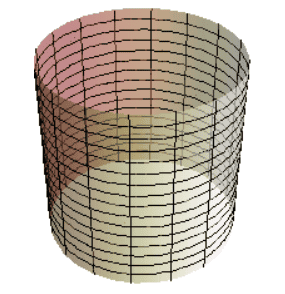This ‘infinite cylinder’ goes on forever, both up and down.

Master the ideas from this section